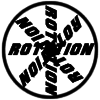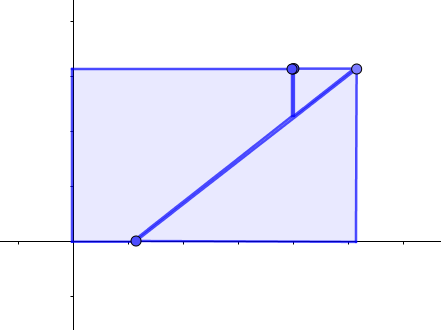#### You may also like### Reflect Again

Follow hints to investigate the matrix which gives a reflection of the plane in the line y=tanx. Show that the combination of two reflections in intersecting lines is a rotation.### Rots and Refs

Follow hints using a little coordinate geometry, plane geometry and trig to see how matrices are used to work on transformations of the plane.### Sine Problem

In this 'mesh' of sine graphs, one of the graphs is the graph of the sine function. Find the equations of the other graphs to reproduce the pattern.

# Squaring the Rectangle

##### Age 14 to 18 Challenge Level:
Here is a rectangle drawn on a set of axes. If the top right corner of the rectangle has coordinates (a, b), how could you calculate the coordinates of the other dots?